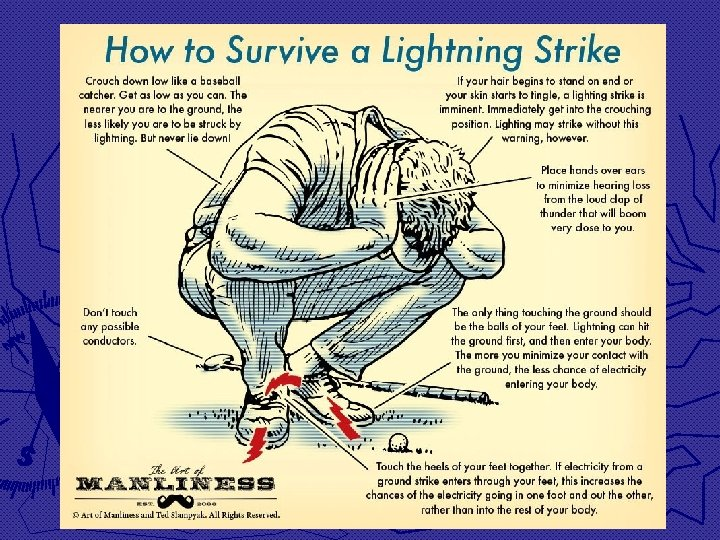# Current Electricity Recall Static Electricity studies excess charges

• Slides: 13Current Electricity Recall Static Electricity studies excess charges making isolated jumps to move away from like charges – high potential; closer to opposite charges – low potential. New Current Electricity studies the continuous, constant flow of charge, again from high to low potential. The main focus of this study is to examine circuits and how they function. ► An Electrical Circuit is a man-made path of conducting materials, usually for the purpose of making an electrical device work. ► And now, “The Story”… keep this scenario in your head as we go through the important physical quantities that are measurable within a circuit…3 Measurable Physical Quantities within a Circuit 1 st Current (I) – rate at which charges flow so eq’n: I = ∆q/∆t in units of amperes (amps or A) where 1 amp = 1 coulomb/sec = 6. 25 x 1018 e-/1 sec (New unit for charge then… amp∙hrs…common on batteries) Important Ideas about Current ► Current does not indicate a net charge in the circuit ► those e- come from the atoms in the conducting material of the various parts of the circuit, NOT pouring in from the wall outlet or battery! ► Charges/Current are not “used up” in a circuit, the #e- entering one end = #e- leaving the other ► these e- simply move from atom to atom, and typically very slowly: ≈ 1 m/hr! (called drift speed)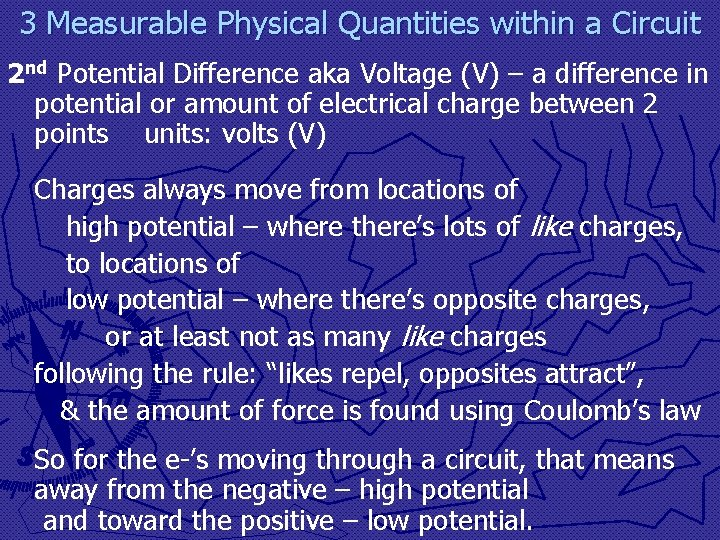3 Measurable Physical Quantities within a Circuit 2 nd Potential Difference aka Voltage (V) – a difference in potential or amount of electrical charge between 2 points units: volts (V) Charges always move from locations of high potential – where there’s lots of like charges, to locations of low potential – where there’s opposite charges, or at least not as many like charges following the rule: “likes repel, opposites attract”, & the amount of force is found using Coulomb’s law So for the e-’s moving through a circuit, that means away from the negative – high potential and toward the positive – low potential.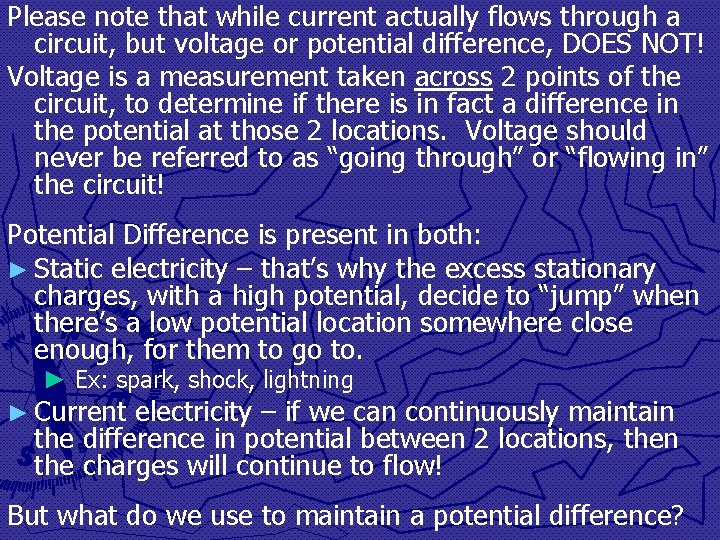Please note that while current actually flows through a circuit, but voltage or potential difference, DOES NOT! Voltage is a measurement taken across 2 points of the circuit, to determine if there is in fact a difference in the potential at those 2 locations. Voltage should never be referred to as “going through” or “flowing in” the circuit! Potential Difference is present in both: ► Static electricity – that’s why the excess stationary charges, with a high potential, decide to “jump” when there’s a low potential location somewhere close enough, for them to go to. ► Ex: spark, shock, lightning ► Current electricity – if we can continuously maintain the difference in potential between 2 locations, then the charges will continue to flow! But what do we use to maintain a potential difference?2 Sources of Potential Difference in a Circuit Current v Time for a Battery ► Batteries § provide DC or direct current – flows in only one direction, at a constant rate § since the location of the high & low potentials of the battery stay fixed § Con: runs out, once all ions reach the low potential (unless rechargeable…) § Pro: portable – no plug needed2 Sources of Potential Difference in a Circuit ► Generators § unless you have your own, we usually tap into a generator at an electrical power plant, by plugging into a wall outlet § Provide AC or alternating current – continually switches direction of travel by 180° § since the locations of the high & low potentials created by the generator alternate positions 120 x’s/sec (60 Hz) § Pro: never runs out, as long as we have fuel to run the generators at the power plants… since e-’s never get to reach the low potential § Con: needs to be connected to generator by cord/plug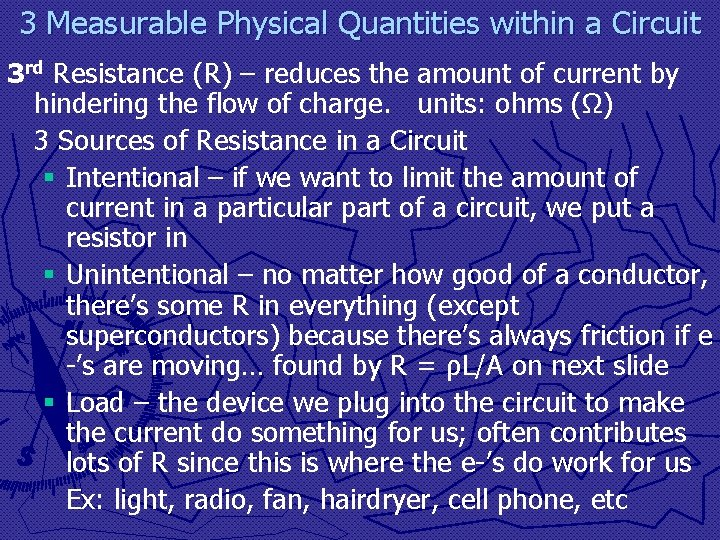3 Measurable Physical Quantities within a Circuit 3 rd Resistance (R) – reduces the amount of current by hindering the flow of charge. units: ohms (Ω) 3 Sources of Resistance in a Circuit § Intentional – if we want to limit the amount of current in a particular part of a circuit, we put a resistor in § Unintentional – no matter how good of a conductor, there’s some R in everything (except superconductors) because there’s always friction if e -’s are moving… found by R = ρL/A on next slide § Load – the device we plug into the circuit to make the current do something for us; often contributes lots of R since this is where the e-’s do work for us Ex: light, radio, fan, hairdryer, cell phone, etc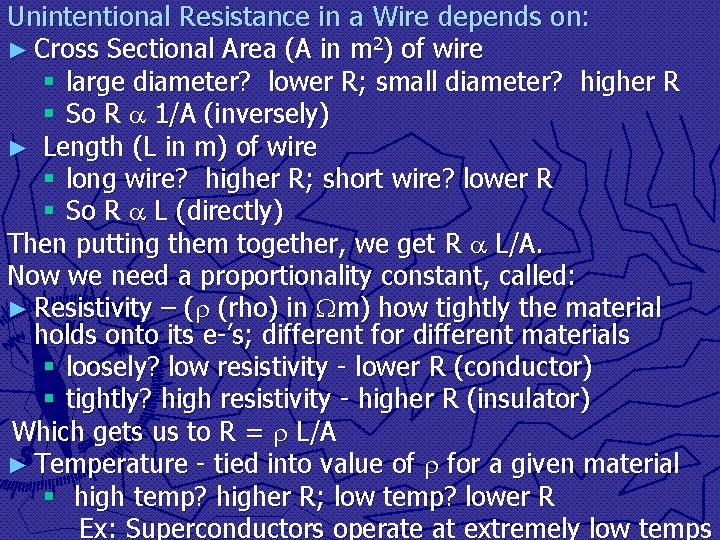Unintentional Resistance in a Wire depends on: ► Cross Sectional Area (A in m 2) of wire § large diameter? lower R; small diameter? higher R § So R 1/A (inversely) ► Length (L in m) of wire § long wire? higher R; short wire? lower R § So R L (directly) Then putting them together, we get R L/A. Now we need a proportionality constant, called: ► Resistivity – ( (rho) in m) how tightly the material holds onto its e-’s; different for different materials § loosely? low resistivity - lower R (conductor) § tightly? high resistivity - higher R (insulator) Which gets us to R = L/A ► Temperature - tied into value of for a given material § high temp? higher R; low temp? lower R Ex: Superconductors operate at extremely low temps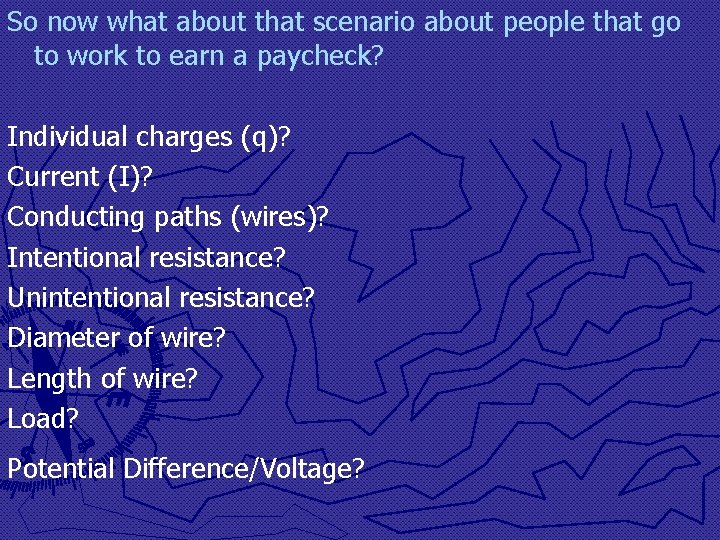So now what about that scenario about people that go to work to earn a paycheck? Individual charges (q)? Current (I)? Conducting paths (wires)? Intentional resistance? Unintentional resistance? Diameter of wire? Length of wire? Load? Potential Difference/Voltage?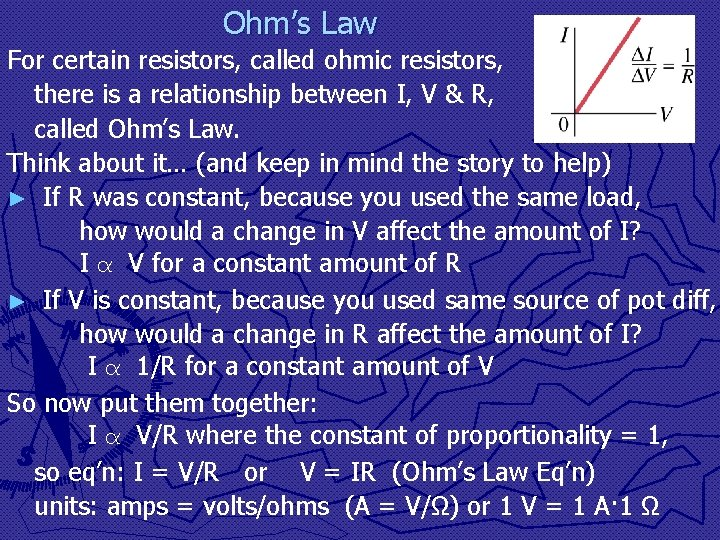Ohm’s Law For certain resistors, called ohmic resistors, there is a relationship between I, V & R, called Ohm’s Law. Think about it… (and keep in mind the story to help) ► If R was constant, because you used the same load, how would a change in V affect the amount of I? I α V for a constant amount of R ► If V is constant, because you used same source of pot diff, how would a change in R affect the amount of I? I α 1/R for a constant amount of V So now put them together: I α V/R where the constant of proportionality = 1, so eq’n: I = V/R or V = IR (Ohm’s Law Eq’n) units: amps = volts/ohms (A = V/Ω) or 1 V = 1 A· 1 Ω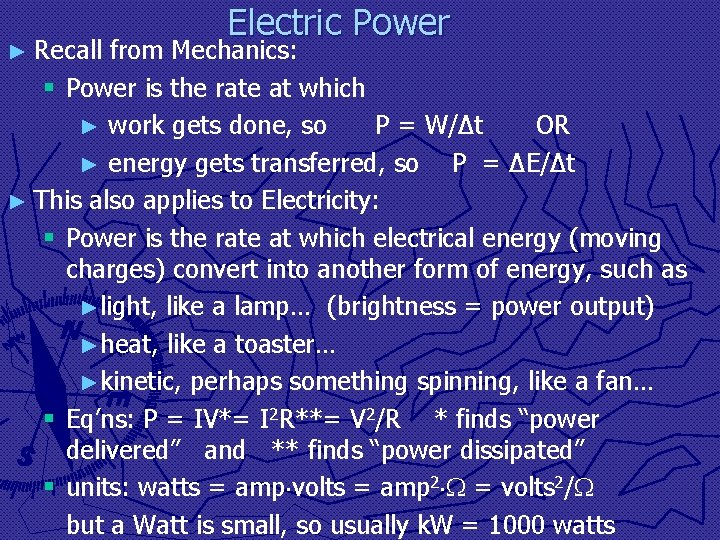► Recall Electric Power from Mechanics: § Power is the rate at which ► work gets done, so P = W/∆t OR ► energy gets transferred, so P = ∆E/∆t ► This also applies to Electricity: § Power is the rate at which electrical energy (moving charges) convert into another form of energy, such as ►light, like a lamp… (brightness = power output) ►heat, like a toaster… ►kinetic, perhaps something spinning, like a fan… § Eq’ns: P = IV*= I 2 R**= V 2/R * finds “power delivered” and ** finds “power dissipated” § units: watts = amp volts = amp 2 = volts 2/ but a Watt is small, so usually k. W = 1000 watts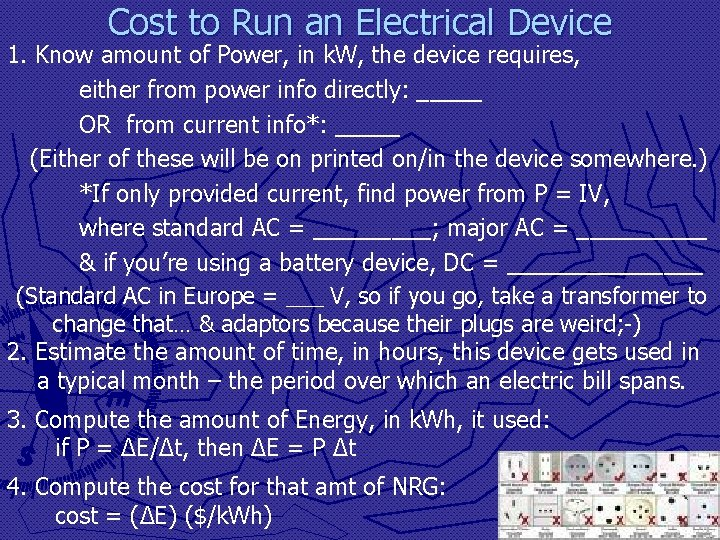Cost to Run an Electrical Device 1. Know amount of Power, in k. W, the device requires, either from power info directly: _____ OR from current info*: _____ (Either of these will be on printed on/in the device somewhere. ) *If only provided current, find power from P = IV, where standard AC = _____; major AC = _____ & if you’re using a battery device, DC = ________ (Standard AC in Europe = ___ V, so if you go, take a transformer to change that… & adaptors because their plugs are weird; -) 2. Estimate the amount of time, in hours, this device gets used in a typical month – the period over which an electric bill spans. 3. Compute the amount of Energy, in k. Wh, it used: if P = ∆E/∆t, then ∆E = P ∆t 4. Compute the cost for that amt of NRG: cost = (∆E) (\$/k. Wh)